# JFET Amplifiers

## Discrete Semiconductor Devices and Circuits

• #### Question 1

 Don’t just sit there! Build something!!

Learning to mathematically analyze circuits requires much study and practice. Typically, students practice by working through lots of sample problems and checking their answers against those provided by the textbook or the instructor. While this is good, there is a much better way.

You will learn much more by actually building and analyzing real circuits, letting your test equipment provide the “answers” instead of a book or another person. For successful circuit-building exercises, follow these steps:

1. Carefully measure and record all component values prior to circuit construction, choosing resistor values high enough to make damage to any active components unlikely.
2. Draw the schematic diagram for the circuit to be analyzed.
3. Carefully build this circuit on a breadboard or other convenient medium.
4. Check the accuracy of the circuit’s construction, following each wire to each connection point, and verifying these elements one-by-one on the diagram.
5. Mathematically analyze the circuit, solving for all voltage and current values.
6. Carefully measure all voltages and currents, to verify the accuracy of your analysis.
7. If there are any substantial errors (greater than a few percent), carefully check your circuit’s construction against the diagram, then carefully re-calculate the values and re-measure.

When students are first learning about semiconductor devices, and are most likely to damage them by making improper connections in their circuits, I recommend they experiment with large, high-wattage components (1N4001 rectifying diodes, TO-220 or TO-3 case power transistors, etc.), and using dry-cell battery power sources rather than a benchtop power supply. This decreases the likelihood of component damage.

As usual, avoid very high and very low resistor values, to avoid measurement errors caused by meter “loading” (on the high end) and to avoid transistor burnout (on the low end). I recommend resistors between 1 kΩ and 100 kΩ.

One way you can save time and reduce the possibility of error is to begin with a very simple circuit and incrementally add components to increase its complexity after each analysis, rather than building a whole new circuit for each practice problem. Another time-saving technique is to re-use the same components in a variety of different circuit configurations. This way, you won’t have to measure any component’s value more than once.

• #### Question 2

This relaxation oscillator circuit uses a resistor-capacitor combination (R1 - C1) to establish the time delay between output pulses:The voltage measured between TP1 and ground looks like this on the oscilloscope display: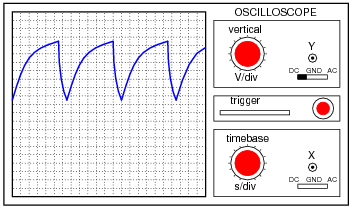A slightly different version of this circuit adds a JFET to the capacitor’s charge current path:Now, the voltage at TP1 looks like this: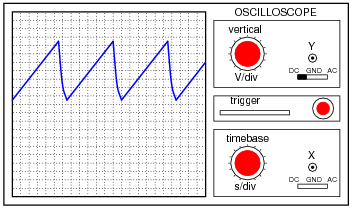What function does the JFET perform in this circuit, based on your analysis of the new TP1 signal waveform? The straight-line charging voltage pattern shown on the second oscilloscope display indicates what the JFET is doing in this circuit.

Hint: you don’t need to know anything about the function of the unijunction transistor (at the circuit’s output) other than it acts as an on/off switch to periodically discharge the capacitor when the TP1 voltage reaches a certain threshold level.

Challenge question: write a formula predicting the slope of the ramping voltage waveform measured at TP1.

• #### Question 3

A student builds this transistor amplifier circuit on a solderless “breadboard”: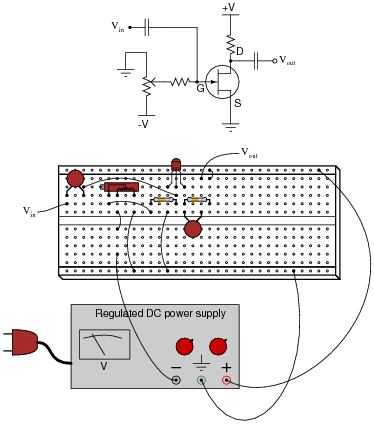The purpose of the potentiometer is to provide an adjustable DC bias voltage for the transistor, so it may be operated in Class-A mode. After some adjustment of this potentiometer, the student is able to obtain good amplification from the transistor (signal generators and oscilloscopes have been omitted from the illustration for simplicity).

Later, the student accidently adjusts the power supply voltage to a level beyond the JFET’s rating, destroying the transistor. Re-setting the power supply voltage back where the student began the experiment and replacing the transistor, the student discovers that the biasing potentiometer must be re-adjusted to achieve good Class-A operation.

Intrigued by this discovery, the student decides to replace this transistor with a third (of the same part number, of course), just to see if the biasing potentiometer needs to be adjusted again for good Class-A operation. It does.

Explain why this is so. Why must the gate biasing potentiometer be re-adjusted every time the transistor is replaced, even if the replacement transistor(s) are of the exact same type?

• #### Question 4

The simple JFET amplifier circuit shown here (built with surface-mount components) employs a biasing technique known as self-biasing: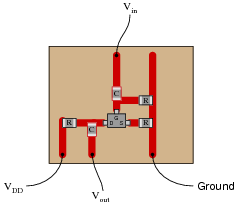Self-biasing provides much greater Q-point stability than gate-biasing. Draw a schematic diagram of this circuit, and then explain how self-biasing works.

• #### Question 5

The voltage gain for a “bypassed” common-emitter BJT amplifier circuit is as follows:Common-source JFET amplifier circuits are very similar:One of the problems with “bypassed” amplifier configurations such as the common-emitter and common-source is voltage gain variability. It is difficult to keep the voltage gain stable in either type of amplifier, due to changing factors within the transistors themselves which cannot be tightly controlled (r′e and gm, respectively). One solution to this dilemma is to “swamp” those uncontrollable factors by not bypassing the emitter (or source) resistor. The result is greater AV stability at the expense of AV magnitude:Write the voltage gain equations for both “swamped” BJT and JFET amplifier configurations, and explain why they are similar to each other.

• #### Question 6

The circuit shown here is a precision DC voltmeter: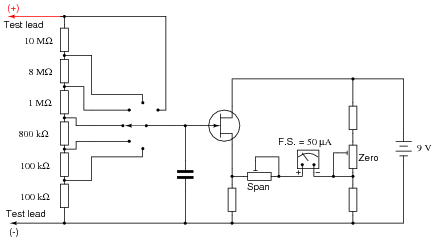Explain why this circuit design requires the use of a field-effect transistor, and not a bipolar junction transistor (BJT).

Explain, step by step, how an increasing input voltage between the test probes causes the meter movement to deflect further.
If the most sensitive range of this voltmeter is 0.1 volts (full-scale), calculate the other range values, and label them on the schematic next to their respective switch positions.
What type of JFET configuration is this (common-gate, common-source, or common-drain)?
What purpose does the capacitor serve in this circuit?
What detrimental effect would result from installing a capacitor that was too large?
Estimate a reasonable value for the capacitor’s capacitance.
Explain the functions of the “Zero” and “Span” calibration potentiometers.
• #### Question 7

This is a schematic of an RF amplifier using a JFET as the active element: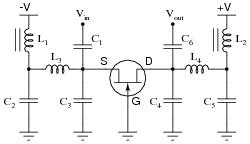What configuration of JFET amplifier is this (common drain, common gate, or common source)? Also, explain the purpose of the two iron-core inductors in this circuit. Hint: inductors L1 and L2 are often referred to as RF chokes.

• #### Question 8

Calculate the approximate input impedance of this JFET amplifier circuit:Explain why it is easier to calculate the Zin of a JFET circuit like this than it is to calculate the Zin of a similar bipolar transistor amplifier circuit. Also, explain how calculation of this amplifier’s output impedance compares with that of a similar BJT amplifier circuit - same approach or different approach?

• #### Question 9

Define what a common-source transistor amplifier circuit is. What distinguishes this amplifier configuration from the other single-FET amplifier configurations, namely common-drain and common-gate? What configuration of BJT amplifier circuit does the common-source FET circuit most resemble in form and behavior?

Also, describe the typical voltage gains of this amplifier configuration, and whether it is inverting or non-inverting.

• #### Question 10

Define what a common-gate transistor amplifier circuit is. What distinguishes this amplifier configuration from the other single-FET amplifier configurations, namely common-drain and common-source? What configuration of BJT amplifier circuit does the common-gate FET circuit most resemble in form and behavior?

Also, describe the typical voltage gains of this amplifier configuration, and whether it is inverting or non-inverting.

• #### Question 11

Define what a common-drain transistor amplifier circuit is. What distinguishes this amplifier configuration from the other single-FET amplifier configurations, namely common-source and common-gate? What configuration of BJT amplifier circuit does the common-drain FET circuit most resemble in form and behavior?

Also, describe the typical voltage gains of this amplifier configuration, and whether it is inverting or non-inverting.

• #### Question 12

Determine whether this amplifier circuit is inverting or non-inverting (i.e. the phase shift between input and output waveforms):Be sure to explain, step by step, how you were able to determine the phase relationship between input and output in this circuit. Also identify the type of amplifier each transistor represents (common-???).

• #### Question 13

It is a well-known fact that temperature affects the operating parameters of bipolar junction transistors. This is why grounded-emitter circuits (with no emitter feedback resistor) are not practical as stand-alone amplifier circuits.

Does temperature affect junction field-effect transistors in the same way, or to the same extent? Design an experiment to determine the answer to this question.

• #### Question 14

Identify what type of amplifier circuit this is, and also what would happen to the output voltage if Vin2 were to become more positive: